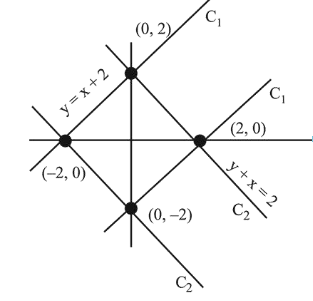# The region represented by

Question:

The region represented by $|x-y| \leq 2$ and $|x+y| \leq 2$ is bounded by a :

1. (1) square of side length $2 \sqrt{2}$ units

2. (2) rhombus of side length 2 units

3. (c) square of area $16 \mathrm{sq}$. units

4. (4) rhombus of area $8 \sqrt{2}$ sq. units

Correct Option: 1

Solution:

Let, $\mathrm{C}_{1}:|y-x| \leq 2$

$\mathrm{C}_{2}:|y+x| \leq 2$

By the diagram, region is squareNow, length of side $=\sqrt{2^{2}+2^{2}}=2 \sqrt{2}$